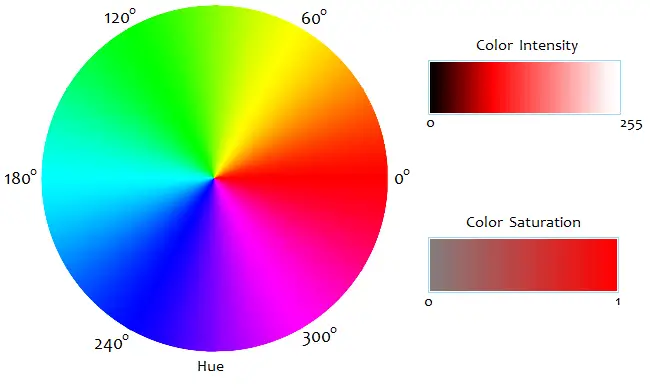# RGB to HSI, HSI to RGB Conversion Calculator

RGB/HSI Conversion Calculator
RGB to HSI

HSI to RGB

Color
RGB values range from 0 to 255. Hue ranges from 0° to 360°. Saturation ranges from 0 to 1. Intensity ranges from 0 to 255.

The RGB color model is an additive system in which each color is defined by the amount of red, green, and blue light emitted. In the RGB scheme, colors are represented numerically with a set of three numbers, each of which ranges from 0 to 255. White has the highest RGB value of (255, 255, 255) while black has the lowest value of (0, 0, 0). This is consistent with the additive nature of the RGB system, since white light is the presence of all colors of light, and black is the absence of all light.

There are other three-parameter representations of colors. One such system is the HSI color model, which encodes colors according to their Hue, Saturation, and Intensity. The HSI model is used by some graphics programs and color monitors as an alternative to, or alongside the RGB representation.

In the HSI system, the hue of a color is its angle measure on a color wheel. Pure red hues are 0°, pure green hues are 120°, and pure blues are 240°. (Neutral colors--white, gray, and black--are set to 0° for convenience.) Intensity is the overall lightness or brightness of the color, defined numerically as the average of the equivalent RGB values.

The HSI definition of saturation is a measure of a color's purity/grayness. Purer colors have a saturation value closer to 1, while grayer colors have a saturation value closer to 0. (In other color models, the meanings and mathematical definitions of "saturation" are slightly different. See HSL and HSV color models for comparison.)### Equations to Convert RGB Values to HSI Values

Suppose R, G, and B are the red, green, and blue values of a color. The HSI intensity is given by the equation

I = (R + G + B)/3.

Now let m be the minimum value among R, G, and B. The HSI saturation value of a color is given by the equation

S = 1 - m/I    if I > 0, or
S = 0            if I = 0.

To convert a color's overall hue, H, to an angle measure, use the following equations:

H = cos-1[ (R - ½G - ½B)/√R² + G² + B² - RG - RB - GB ]            if G ≥ B, or
H = 360 - cos-1[ (R - ½G - ½B)/√R² + G² + B² - RG - RB - GB ]    if B > G,

where the inverse cosine output is in degrees.

### Equations to Convert HSI Values to RGB Values

To convert hue, saturation, and intensity to a set of red, green, and blue values, you must first note the value of H. If H = 0, then R, G, and B are given by

R = I + 2IS
G = I - IS
B = I - IS.

If 0 < H < 120, then

R = I + IS*cos(H)/cos(60-H)
G = I + IS*[1 - cos(H)/cos(60-H)]
B = I - IS.

If H = 120, then the red, green, and blue values are

R = I - IS
G = I + 2IS
B = I - IS.

If 120 < H < 240, then

R = I - IS
G = I + IS*cos(H-120)/cos(180-H)
B = I + IS*[1 - cos(H-120)/cos(180-H)].

If H = 240 then

R = I - IS
G = I - IS
B = I + 2IS.

And if 240 < H < 360, we have

R = I + IS*[1 - cos(H-240)/cos(300-H)]
G = I - IS
B = I + IS*cos(H-240)/cos(300-H).